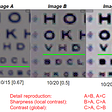# Superpixels and SLIC

A superpixel can be defined as a group of pixels that share common characteristics (like pixel intensity ). Superpixels are becoming useful in many Computer Vision and Image processing algorithms like Image Segmentation, Semantic labeling, Object detection and tracking etc because of the following-

• Superpixels have a perceptual meaning since pixels belonging to a given superpixel share similar visual properties.
• They provide a convenient and compact representation of images that can be very useful for computationally demanding problems.

## Superpixels in the context of Image Segmentation

Image Segmentation is the process of partitioning an image into multiple segments(set of pixels or superpixels). The goal is to represent the image as something that is more meaningful and easier to analyze. In other words, image segmentation is the process of assigning a label to every pixel in an image such that pixels with the same label share certain characteristics. Segmentation can be used to locate objects and boundaries (lines, curves etc.) in images. Some of its practical applications are in -

• Medical Imaging
• Object detection (Face, pedestrian detection)
• Recognition Tasks (Face, Fingerprint recognition)
• Video Surveillance etc.

## SLIC (Simple Linear Iterative Clustering) Algorithm for Superpixel generation

This algorithm generates superpixels by clustering pixels based on their color similarity and proximity in the image plane. This is done in the five-dimensional [labxy] space, where [lab] is the pixel color vector in CIELAB color space and xy is the pixel position. We need to normalize the spatial distances in order to use the Euclidean distance in this 5D space because the maximum possible distance between two colors in the CIELAB space is limited whereas the spatial distance in the xy plane depends on the image size. Therefore, In order to cluster pixels in this 5D space, a new distance measure that considers superpixel size was introduced which is described below.

Distance Measure

Some useful notations-

This algorithm takes as input a desired number of approximately equally-sized superpixels K. At the onset of the algorithm, K superpixel cluster centers Cₖ= [lₖ, aₖ, bₖ, xₖ, yₖ] are chosen with k= [1,K] at regular grid intervals S. Since the spatial extent of any superpixel is approximately S²(the approximate area of a super-pixel), it is safely assumed that pixels that are associated with this cluster center lie within a 2S×2S area around the superpixel center on the xy plane. The normalized distance measure (Dₛ) to be used in the 5D space is defined as :

Dₛ = dₗₐᵦ +( m/S)*dₓᵧ …. (eq 1)

where dₗₐᵦ = √((lₖ−lᵢ)²+ (aₖ−aᵢ)²+ (bₖ−bᵢ)²), dₓᵧ = √((xₖ−xᵢ)²+ (yₖ−yᵢ)²) and Dₛ is the sum of the lab distance (dₗₐᵦ)and the xy plane distance (dₓᵧ) normalized by the grid interval S. A variable m is introduced in Dₛ allowing us to control the compactness of a superpixel. The greater the value of m, the more compact the cluster. This value can be in the range [1,20].

Algorithm

This algorithm begins by sampling K regularly spaced cluster centers and moving them to seed locations corresponding to the lowest gradient position in a 3×3 neighborhood (This is done to avoid placing them at an edge and to reduce the chances of choosing a noisy pixel). Image gradients are computed as:

G(x,y) = ‖I(x+ 1,y)−I(x−1,y)‖²+‖I(x,y+ 1)−I(x,y−1)‖²

where I(x,y) is the lab vector corresponding to the pixel at position (x,y), and ‖.‖ is the L2 norm. This takes into account both color and intensity information. Each pixel in the image is associated with the nearest cluster center whose search area overlaps this pixel. After all the pixels are associated with the nearest cluster center, a new center is computed as the average labxy vector of all the pixels belonging to the cluster.

The process of associating pixels with the nearest cluster center and recomputing the cluster center is repeated until convergence. At the end of this process, a few stray labels may remain, that is, a few pixels in the vicinity of a larger segment having the same label but not connected to it. Connectivity can be enforced in the last step of the algorithm by relabeling disjoint segments with the labels of the largest neighboring cluster.

SLIC Algorithm can be summarized as-

My SLIC algorithm implementation in python can be found in the GitHub Repository. Some of the results of my implementation (with K = 100 and m = 20 )-

References

 https://github.com/BIDS/BSDS500 (For Image Segmentation Dataset)

--

--

--

## More from Darshita Jain

Researcher at Tata Research Development and Design Centre| MTech CSE | IIT Gandhinagar

Love podcasts or audiobooks? Learn on the go with our new app.

## Research Papers On Minimum Spanning Tree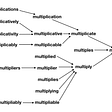## What Borges Can Tech Us About Overfitting and Underfitting in Deep learning Models## Natural Language Processing(NLP)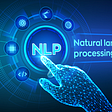## The Machine Learning Architecture Powering LinkedIn Recruiter## Neural Network model for Answer selection## What did I learn from my first Kaggle competition?## Building an End to End Search Engine Chatbot for the website using Amazon Lex, Google Knowledge## Darshita Jain

Researcher at Tata Research Development and Design Centre| MTech CSE | IIT Gandhinagar

## SqueezeNets and MobileNets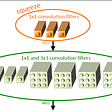## Instance segmentation has become one of the most significant, complex and challenging fields in…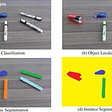## Detail reproduction as a key to object detection